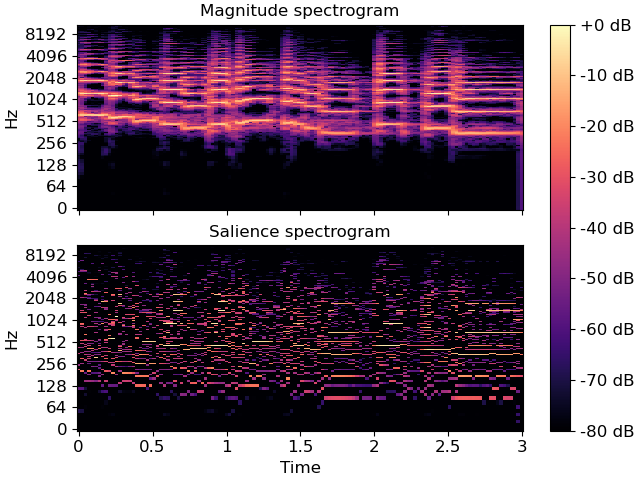# librosa.salience¶

librosa.salience(S, freqs, h_range, weights=None, aggregate=None, filter_peaks=True, fill_value=nan, kind='linear', axis=0)[source]

Harmonic salience function.

Parameters
Snp.ndarray [shape=(d, n)]

input time frequency magnitude representation (e.g. STFT or CQT magnitudes). Must be real-valued and non-negative.

freqsnp.ndarray, shape=(S.shape[axis])

The frequency values corresponding to S’s elements along the chosen axis.

h_rangelist-like, non-negative

Harmonics to include in salience computation. The first harmonic (1) corresponds to `S` itself. Values less than one (e.g., 1/2) correspond to sub-harmonics.

weightslist-like

The weight to apply to each harmonic in the summation. (default: uniform weights). Must be the same length as `harmonics`.

aggregatefunction

aggregation function (default: np.average)

If `aggregate=np.average`, then a weighted average is computed per-harmonic according to the specified weights. For all other aggregation functions, all harmonics are treated equally.

filter_peaksbool

If true, returns harmonic summation only on frequencies of peak magnitude. Otherwise returns harmonic summation over the full spectrum. Defaults to True.

fill_valuefloat

The value to fill non-peaks in the output representation. (default: np.nan) Only used if `filter_peaks == True`.

kindstr

Interpolation type for harmonic estimation. See `scipy.interpolate.interp1d`.

axisint

The axis along which to compute harmonics

Returns
S_salnp.ndarray, shape=(len(h_range), [x.shape])

`S_sal` will have the same shape as `S`, and measure the overal harmonic energy at each frequency.

Examples

```>>> y, sr = librosa.load(librosa.ex('trumpet'), duration=3)
>>> S = np.abs(librosa.stft(y))
>>> freqs = librosa.fft_frequencies(sr)
>>> harms = [1, 2, 3, 4]
>>> weights = [1.0, 0.5, 0.33, 0.25]
>>> S_sal = librosa.salience(S, freqs, harms, weights, fill_value=0)
>>> print(S_sal.shape)
(1025, 115)
>>> import matplotlib.pyplot as plt
>>> fig, ax = plt.subplots(nrows=2, sharex=True, sharey=True)
>>> librosa.display.specshow(librosa.amplitude_to_db(S, ref=np.max),
...                          sr=sr, y_axis='log', x_axis='time', ax=ax)
>>> ax.set(title='Magnitude spectrogram')
>>> ax.label_outer()
>>> img = librosa.display.specshow(librosa.amplitude_to_db(S_sal,
...                                                        ref=np.max),
...                                sr=sr, y_axis='log', x_axis='time', ax=ax)
>>> ax.set(title='Salience spectrogram')
>>> fig.colorbar(img, ax=ax, format="%+2.0f dB")
```